# 这些堪称经典的公式，马上要和你古德拜

1、一对多查询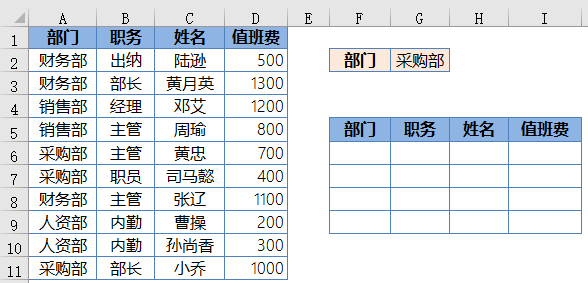F6单元格输入以下数组公式，按住SHift+ctrl不放按回车，然后把公式向下向右拖动。

=INDEX(A:A,SMALL(IF(\$A\$2:\$A\$11=\$G\$2,ROW(\$2:\$11),4^8),ROW(A1)))&””

=FILTER(A1:D11,A1:A11=G2)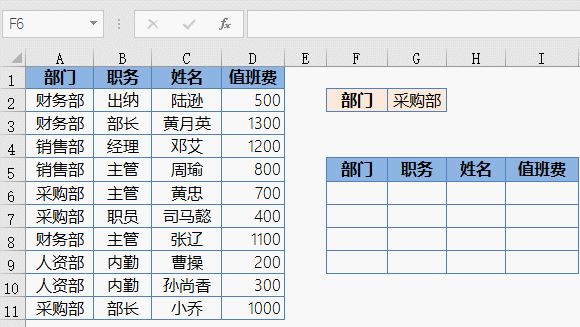2、多对多查询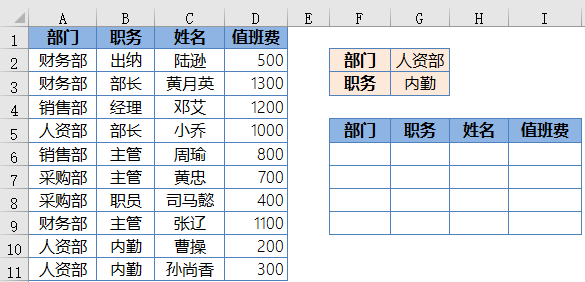F6单元格输入以下数组公式，按住SHift+ctrl不放按回车，然后把公式向下向右拖动。

=INDEX(A:A,SMALL(IF((\$A\$2:\$A\$11=\$G\$2)*(\$B\$2:\$B\$11=\$G\$3),ROW(\$2:\$11),4^8),ROW(A1)))&””

=FILTER(A1:D11,A1:A11&B1:B11=G2&G3)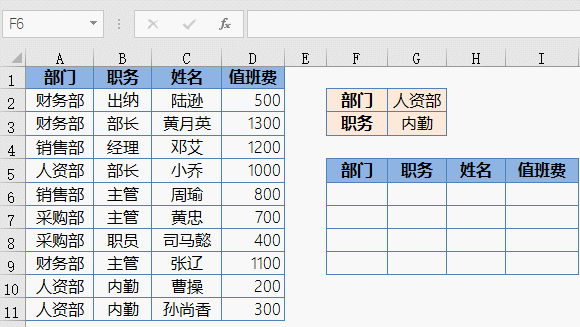3、一列转多列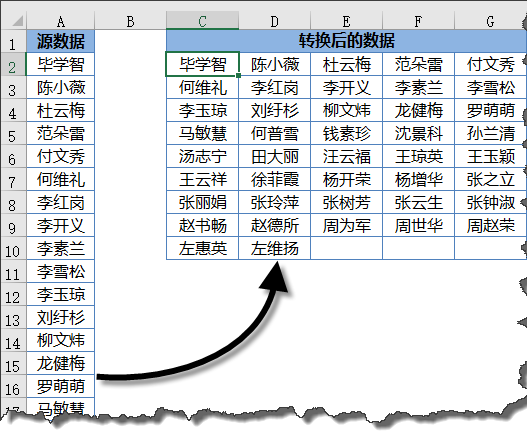C2单元格输入以下公式，然后把公式向下向右拖动到出现空白为止。

=INDEX(\$A:\$A,ROW(A1)*5-4+COLUMN(A1))&””

=INDEX(A:A,SEQUENCE(11,5,2))&””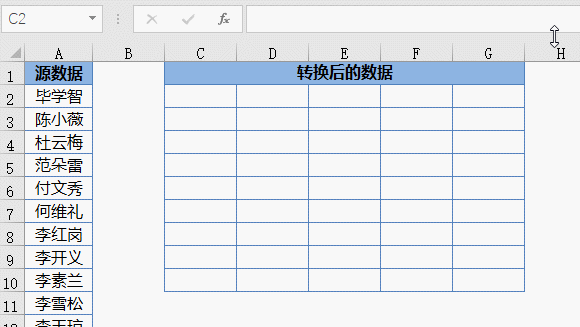4、提取不重复清单G2单元格输入以下数组公式，按住SHift+ctrl键不放按回车，然后把公式向下向右拖动到出现空白为止。

=INDEX(B:B,MATCH(0,COUNTIF(G\$1:G1,B\$2:B\$76),0)+1)&””

=UNIQUE(B2:B75)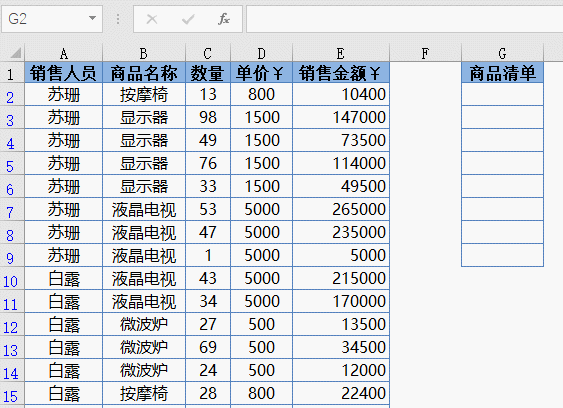5、提取符合条件的不重复记录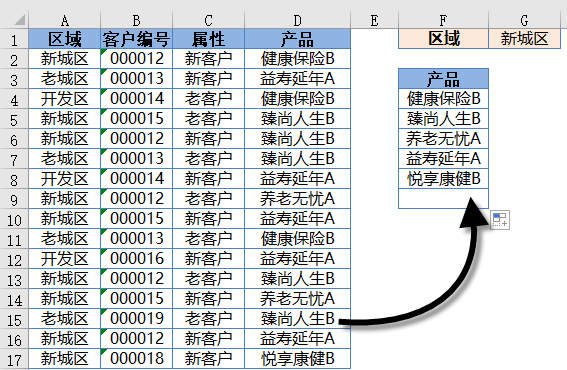F4单元格输入以下数组公式，按住SHift+ctrl键不放按回车，然后把公式向下拖动到出现空白为止。

=INDEX(D:D,SMALL(IF((MATCH(A\$2:A\$17&D\$2:D\$17,A:A&D:D,)=ROW(\$2:\$17))*(A\$2:A\$17=G\$1),ROW(\$2:\$17),4^8),ROW(A1)))&””

=UNIQUE(FILTER(D2:D17,A2:A17=G1))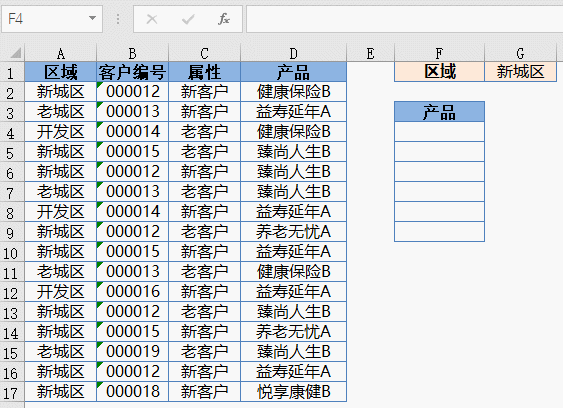https://pan.baidu.com/s/1zf1CYPwFkPpEN57_5WD2Qg